# Texas Go Math Kindergarten Module 12 Assessment Answer Key

Refer to our Texas Go Math Kindergarten Answer Key Pdf to score good marks in the exams. Test yourself by practicing the problems from Texas Go Math Kindergarten Module 12 Assessment Answer Key.

## Texas Go Math Kindergarten Module 12 Assessment Answer Key

Concepts and Skills

DIRECTIONS: 1. Four children are sitting together. Then one child leaves. Complete the subtraction sentence. (TEKS K.3.A) 2. There are 5 children at a playground. Three children leave. How many children are there now? Write the subtraction sentence. (TEKS K.3.A) 3. There are 4 seahorses. Three seahorses swim away. How many seahorses are left? Complete the subtraction sentence. (TEKS K.3.B)

Question 1.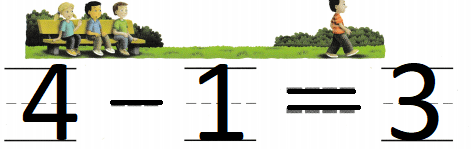Explanation:
Four children are sitting together.
Then one child leaves.
4 – 1 = 3
so, 3 children left.

Question 2.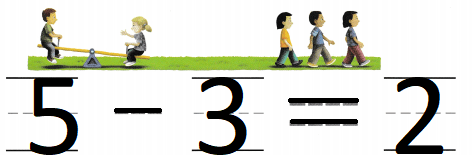Explanation:
There are 5 children at a playground.
Three children leave.
5 – 3 = 2
so, 2 children left in the playground

Question 3.Explanation:
There are 4 seahorses.
Three seahorses swim away.
4 – 3 = 1
so, 1 sea horse is left.

DIRECTIONS: 4. There are 5 fish. Two fish swim away. How many fish are left? Circle and mark an X to show the fish that swim away. Complete the subtraction sentence. (TEKS K.3.B) 5. There are 3 birds. Two birds fly away. How many birds are left? Circle and mark an X to show the birds that leave. Complete the subtraction sentence. (TEKS K.3.B ) 6. Choose the correct answer. There are 4 children on the swings. Then 2 children leave. Act out the problem. What does the picture show? (TEKS K.3.C)

Question 4.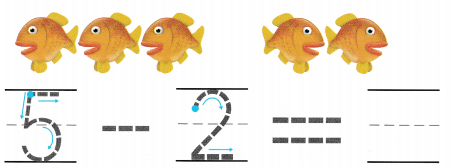Explanation:
There are 5 fish.
Two fish swim away.
5 – 2 = 3
so, 3 fish are left

Question 5.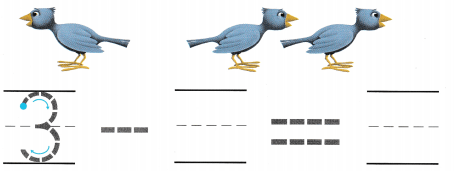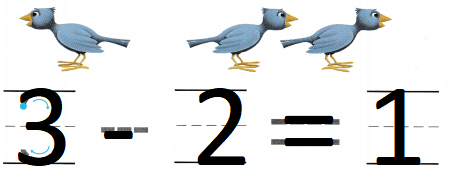Explanation:
There are 3 birds.
Two birds fly away.
3 – 2 = 1
1  birds are left

Question 6.
Texas Test Prep